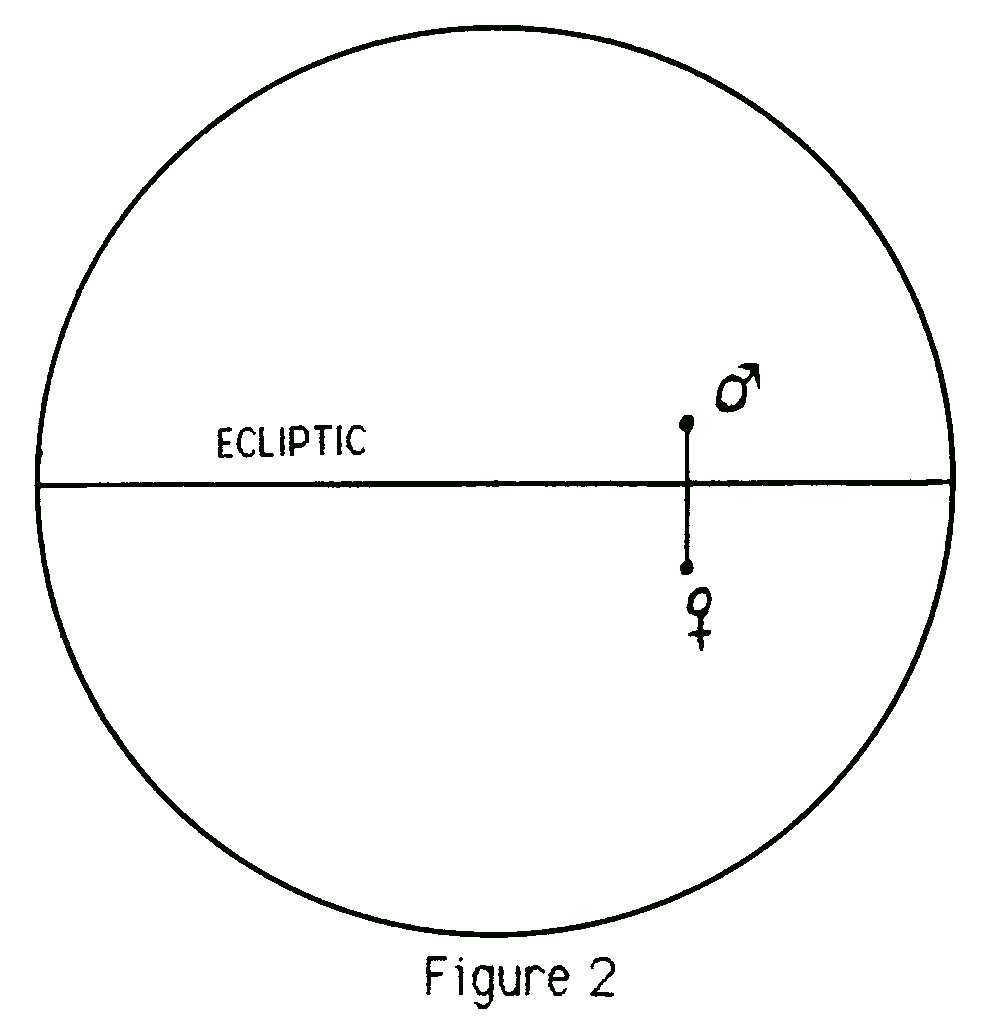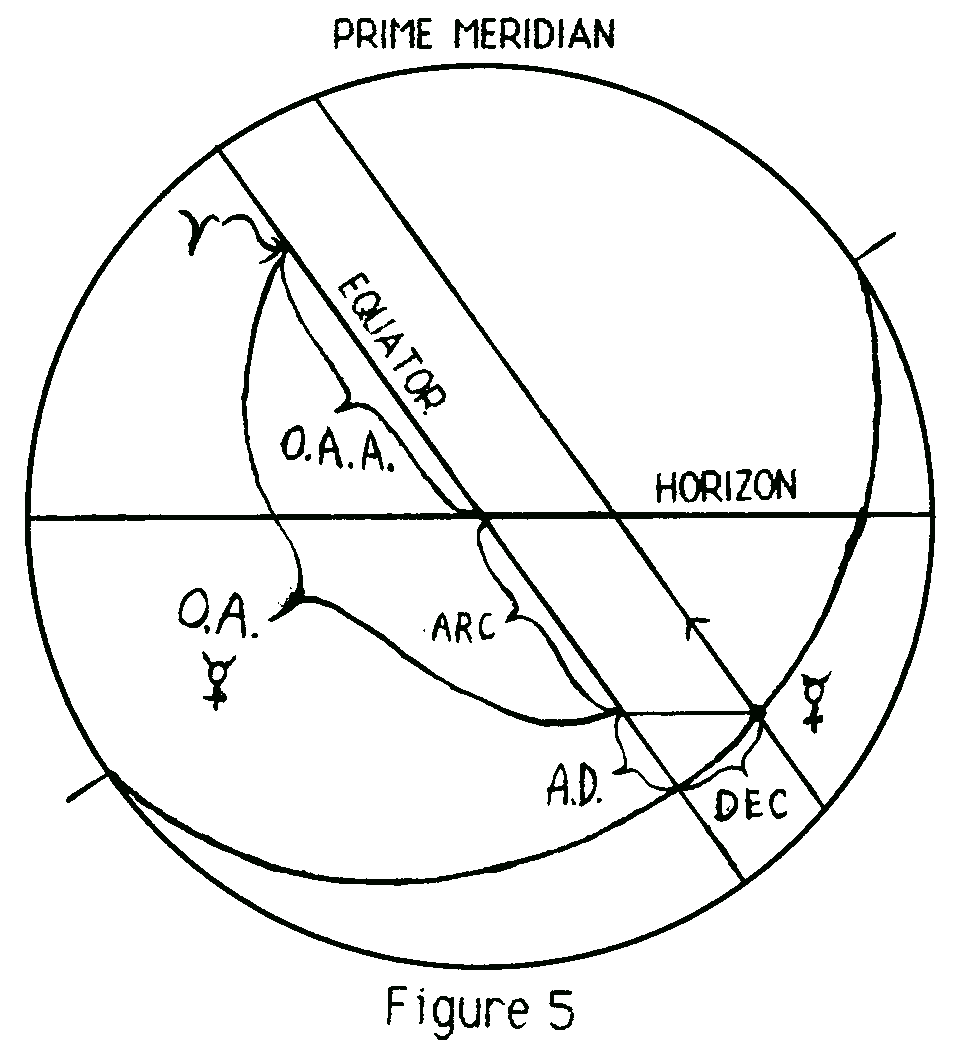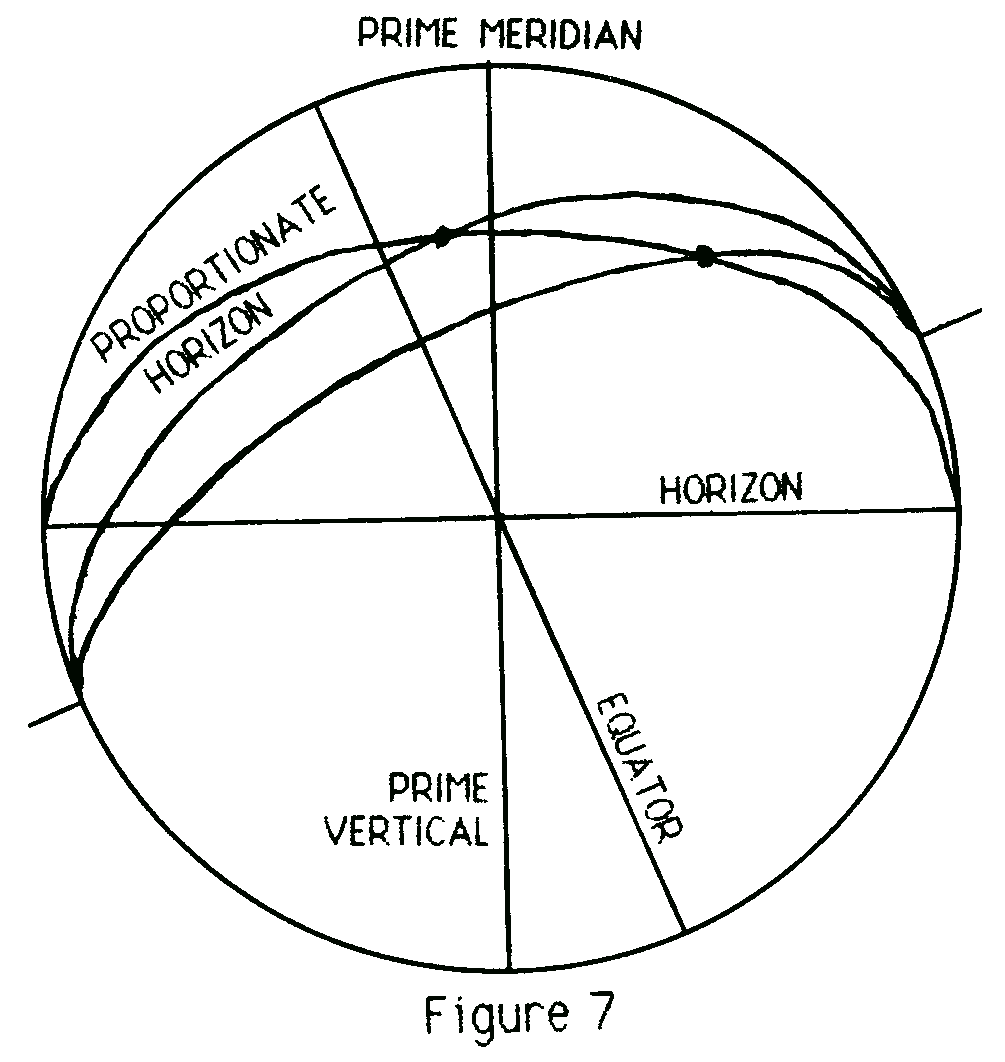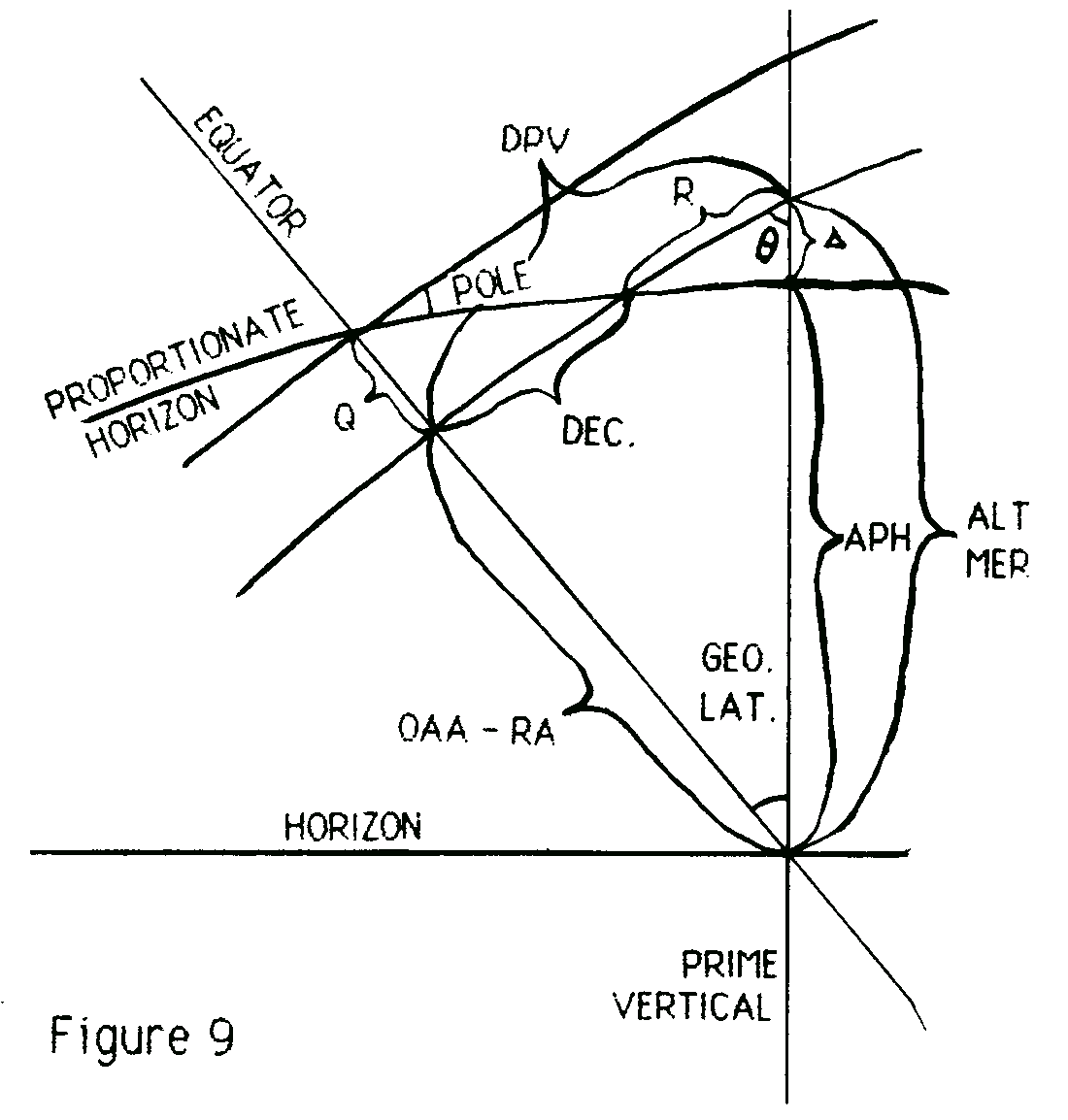# Primary Directions

## Rique Pottenger

Recently Astro Computing Services, where I work as a programmer, decided to add a report which unified all the forms of progressions, directions, and transits into one. In the UPDATE (Unified Progressed, Directed And Transiting Events) report, everything that happens in a given time period is shown together, so people don't have to look through several different reports.

In the course of programming this report, I had to do some research into primary directions. I found that the way that they had been done at ACS was theoretically incorrect. The original programmer had used the method of DeLuce as given in The Complete Method of Prediction. But as Charles Jayne states in his booklet Progressions and Directions, that method is wrong. DeLuce's method is equivalent to Alan Leo's, which I regret to say that my brother Mark and I have been using. [Editor's note: this has been corrected as of June 1987.] Neither author states anywhere that their method is only approximately correct, which is the case.

I can understand why Leo's and DeLuce's methods were used, since it is much more tedious to calculate the speculum with the correct formulae. Fortunately, we live in an age of computers.

Rather than just plunge into the specifics of the differences, I am going to give a full explanation of primary directions for those who are not familiar with this method of prediction.

To start with, primary directions are aspects formed in Ascension (i.e., measured along the Equator rather than the Ecliptic). They are formed during the first hours of birth as the planets are carried up in the sky from their birth positions by the rotation of the Earth. Figure 1 is a projection of the celestial sphere onto the Prime Meridian, showing how a planet is carried along an arc parallel to the Equator as the sphere (actually the Earth) rotates.The complexity in primary directions arises from the decision as to when planets are in aspect. The definition of an aspect is different in primary directions from methods of prediction using zodiacal coordinates. The normal way of measuring aspects in zodiacal longitude is merely along the Ecliptic—arcs perpendicular to the Ecliptic are drawn from the bodies to the Ecliptic, and the distance between the Ecliptic intersections is the aspect angle. Thus bodies may be considered conjunct even though there is considerable distance between them in latitude. Figure 2 shows this situation. Mars is a few degrees north of the Ecliptic, Venus a few degrees south, but since their zodiacal longitudes are identical, they are considered conjunct.In primary directions, however, two planets are considered conjunct when they are on the same horizon. To explain further, consider the Ascendant. It is the intersection of the Ecliptic with the Horizon of the chart (in the East). Figure 3 shows the Sun on the Horizon.[Note: see correction in Sag 1987 issue.]Figure 4 adds the Ecliptic, a meridian through the Sun, and several labels. The Right Ascension of a planet is the distance along the Equator from the 0 Aries point to the point intersected by a meridian through the planet. The Declination is the distance along the meridian of the planet from the Equator to the planet. The angle marked CL between the horizon and Equator is the co-latitude. That is, the geographic latitude subtracted from 90 degrees. The arc along the Equator from the horizon to the planet's meridian is called the Ascensional Difference (abbreviated as A.D. in the diagram). By standard spherical trigonometry, its formula is:

sine(A.D.) = tan(declination) / tan(co-latitude)

or, since tan(latitude) = 1 / tan(co-latitude),

sine(A.D.) = tan(declination) x tan(latitude).

If declination and geographic latitude are taken as signed numbers (positive for north, negative for south) this formula will give a correct (signed) value automatically, with no need for checking four possibilities as in Leo or DeLuce.

Now the Right Ascension of the planet minus the Ascensional Difference gives the Oblique Ascension, the distance along the Equator from the 0 Aries point to the intersection of the Horizon and the Equator when the body is on the Horizon. This calculation is just as valid when the planet is not on the Horizon at birth, and in that case the distance between the oblique ascension of the planet and the oblique ascension of the Ascendant is the arc of direction for when the planet will be conjunct the Ascendant. Figure 5 shows such a case. Note that the planet is carried around on a small circle parallel to the Equator.So when is a directed planet conjunct a natal planet? When it is on the natal planet's proportionate horizon. The proportionate horizon is a great circle perpendicular to the prime vertical (as is the horizon of the chart) which passes through the body, and through the intersections of the horizon and the prime meridian. Figure 6 shows a proportionate horizon. It is just the horizon of the chart rotated on its north-south axis until it intersects the planet. Figure 7 shows two planets on the same proportionate horizon. Meridians have been drawn to show that their right ascensions are not the same.How then does one tell when bodies are on the same proportionate horizon? A procedure similar to that for finding the oblique ascension is carried out. The distance along the Equator from 0 Aries to the intersection of the Equator and the proportionate horizon of the body is found (that distance will be called Horizontal Ascension in this article). If these distances are the same for two planets, then they must be on the same proportionate horizon. Figure 8 shows a planet on its proportionate horizon with meridians drawn through both the planet and the intersection of the Equator with the planet's proportionate horizon. The angle between the meridian and the proportionate horizon at the Equator is called the planet's own pole. When the planet is on the horizon, its proportionate horizon coincides with the horizon of the chart, and its pole is the geographic latitude. When the planet is on the prime meridian, its own pole is zero.The distance along the Equator from the proportionate horizon to the meridian of the planet is called the little ascensional difference, or the modified ascensional difference. It is commonly abbreviated as Q in books on primary directions. Like the use of the A.D. earlier, subtract Q from the Right Ascension to get the Horizontal Ascension. The angle between the proportionate horizon and the Equator labeled CP in Figure 8 is the co-pole—90 degrees minus the pole. From spherical trigonometry,

sine(Q) = tan(declination)/tan(co-pole)

or

sine(Q) = tan(declination) x tan(pole).

So now the question is, how are Q and the pole to be found? This is where the difference in method appears.

First, more definitions. The distance along the Equator from the Prime Meridian to the meridian of a planet is its Meridian Distance. The ascensional difference of a planet plus 90 degrees is its Semi-Arc. DeLuce states that the proportion between the Meridian Distance of a body and the Semi-Arc of that body is equal to the proportion between the modified ascensional difference and the ascensional difference of the body. From the modified ascensional difference, he computes the pole. As a formula:

Meridian Distance / Semi-Arc = Q / A.D.

If there is no alternate way known to find Q or the pole, this must be accepted and used. But Jayne gives one that is demonstrably trigonometrically correct. Consider Figure 9.Again a planet is shown on its proportionate horizon with meridians through it and the intersection of its proportionate horizon with the Equator, but several subsidiary arcs and angles have been labeled. Start with the distance along the Equator from the planet's meridian to the horizon of the chart. This is the oblique ascension of the ascendant minus the right ascension of the planet, and is labeled OAA - RA on the diagram. From this value and the geographic latitude, the altitude of the planet's meridian (distance from the meridian to the horizon of the chart along the prime vertical) is derived by:

tan(ALT MER) = tan(OAA-RA) / cosine(latitude).

The third side of that particular spherical triangle (labeled DPV on the figure) is given by:

tan(DPV) = tan(latitude) x sine(OAA-RA)

and the other angle (labeled theta) by:

cosine(theta) = cosine(OAA-RA) x sine(latitude).

Now DPV minus the declination gives R, the distance along the planet's meridian from its proportionate horizon to the prime vertical. From R and theta the distance delta can be found:

tan(delta) = cosine(theta) x tan(R).

Finally, the altitude of the meridian minus delta gives APH, the altitude of the proportionate horizon. Switching to the triangle with sides on the prime vertical, Equator, and proportionate horizon, the co-pole can now be found:

cosine(co-pole) = cosine(APH) x sine(latitude)

or, since the pole is the complement of the co-pole,

sine(pole) = cosine(APH) x sine(latitude).

Once the pole is found, the formula several paragraphs above gives Q, which gives the Horizontal Ascension.

Now the proportion formula can be tested. Take as an example,

Right Ascension = 30 degrees

Obl. Asc. of Asc = 60 degrees

geo. latitude = 40 degrees

Declination = 10 degrees

Then:

Ascensional Difference = 8 d 30 minutes

Semi-Arc = 98 degrees 30 minutes

Meridian Distance = 60 degrees

Little Ascensional Difference = 5 d 11 m (according to DeLuce).

pole = 27 degrees 7.5 minutes (from that Q)

OAA - RA = 30 degrees

altitude of meridian = 37 degrees

DPV = 22 degrees 45.6 minutes

theta = 56 degrees 10.45 minutes

R = 12 degrees 45.6 minutes

delta = 7 degrees 11 minutes

APH = 29 degrees 49 minutes

pole = 33 degrees 53.7 minutes

Little Ascensional Difference = 6 d 48.2 m (correct value).

As you can see, there is considerable difference. I am sure that the proportion method descends from the earliest days of Astrology, when that sort of calculation was all that the mathematical tools would support. But it should not be used when there are computers available to do it right.

I have left a great deal out of this article, notably how to form aspects other than conjunctions, how to handle celestial latitude, and how to translate an arc into time in the life. If there is interest in more information, let the editor know and I will carry on.